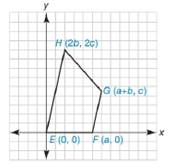Chapter 10.2, Problem 41EElementary Geometry For College St...

7th Edition
Alexander + 2 others
ISBN: 9781337614085

Solutions

Chapter
SectionElementary Geometry For College St...

7th Edition
Alexander + 2 others
ISBN: 9781337614085
Textbook Problem

Quadrilateral E F G H has the vertices E 0 ,   0 , F a ,   0 , G a + b ,   c , and H 2 b , 2 c . Verify that E F G H is a trapezoid by showing that the slopes of two sides are equal.To determine

To prove:

The quadrilateral EFGH is trapezoid by showing the slopes of two sides are equal.Explanation

To prove that the quadrilateral is a trapezoid, one of the opposite sides must be parallel,

By theorem,

If two lines are parallel, then their slopes are equal.

(i.e) If l1l2, then m1=m2.

So we can prove this by using the slopes.

Thus we have to prove the line EH is parallel to the line FG.

The slope of the line that contains the points x1,y1 and x2,y2 is given by

m=y2-y1x2-x1 for x2x1

Let mEH- and mFG- are the slopes of the line EH- and FG- respectively.

The given vertices are E0, 0, and H2b, 2c.

Using the slope formula and choosing x1=0, x2=2b, y1=0, and y2=2c

Still sussing out bartleby?

Check out a sample textbook solution.

See a sample solution

The Solution to Your Study Problems

Bartleby provides explanations to thousands of textbook problems written by our experts, many with advanced degrees!

Get Started

Find more solutions based on key concepts